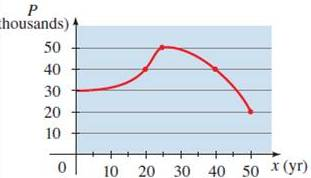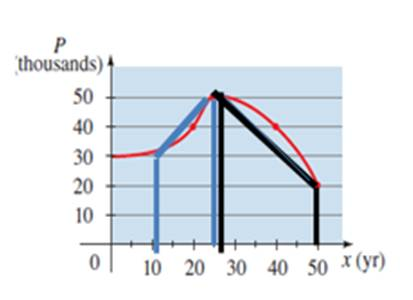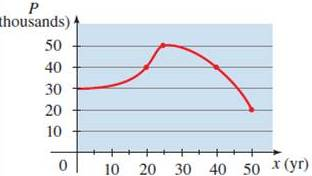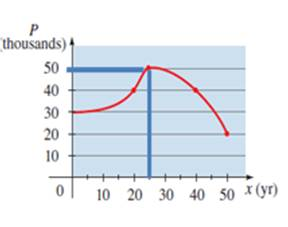# the intervals on which function is increasing or decreasing### Precalculus: Mathematics for Calcu...

6th Edition
Stewart + 5 others
Publisher: Cengage Learning
ISBN: 9780840068071### Precalculus: Mathematics for Calcu...

6th Edition
Stewart + 5 others
Publisher: Cengage Learning
ISBN: 9780840068071

#### Solutions

Chapter 2.3, Problem 48E

a.

To determine

## the intervals on which function is increasing or decreasing

Expert Solution

The function is increasing on 10yr25yr and decreasing on 25yr50yr

### Explanation of Solution

Given information : The provided graph shown belowAt the interval 10yr25yr the graph interval is increasing

At the interval 25yr50yr the graph interval is decreasing which is shown in figure

b.

To determine

Expert Solution

### Explanation of Solution

Given information : The provided graph shown belowThe maximum population is 50,000 which is attain in 1975

### Have a homework question?

Subscribe to bartleby learn! Ask subject matter experts 30 homework questions each month. Plus, you’ll have access to millions of step-by-step textbook answers!